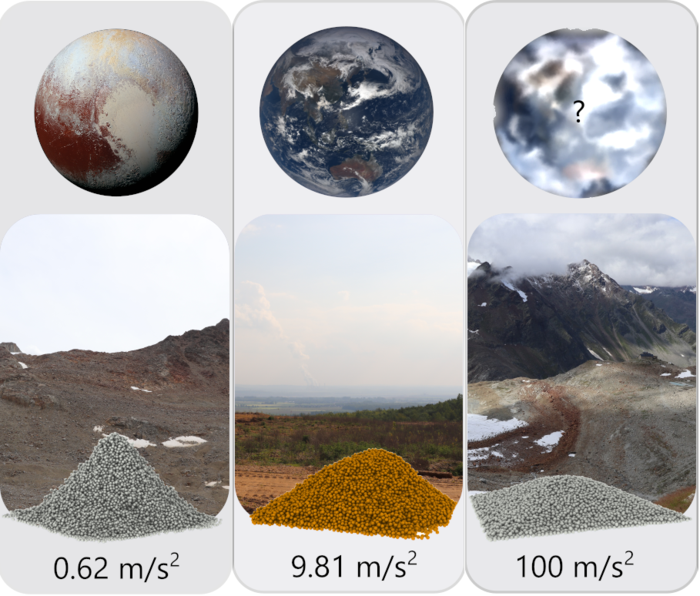News Release

# Model to predict the angle of repose of sand hills developed

Peer-Reviewed Publication

University of Cologneimage: Simulated angles of response on Pluto (lelft), Earth (middle) and on a hypothedical planet (right). Each hill would be shaped differently according to the planet's gravity and the granule size of the material. view more  Credit: Filip Elekes

The prediction of the angle of repose for raised mounds of dry cohesive granular material is a long-standing problem in the natural sciences and in industry. Using numerical (particle-based) simulations, geophysicists Dr Eric Parteli (until 2020 at the University of Cologne, now at the University of Duisburg-Essen) and Filip Elekes (University of Cologne) have developed a mathematical model that allows them to predict this angle, hence solving a long-standing problem.
Many people will remember playing in the sandbox as a child: If you let sand trickle down, it forms a sand cone that looks slightly different depending on the size of the sand grains. ‘In physics, what is most interesting in this regard is the angle of the cone created by the flowing sand – the so-called angle of repose,’ said Filip Elekes, first author of the paper now published in PNAS. ‘The angle of repose represents a measure of the flowability of the granules: The more flowable the granules, the smaller the angle of repose.’
The interplay of particle size, flow behaviour (angle of repose) and gravity (gravitational acceleration) is important for various industrial applications, such as additive manufacturing (e.g., 3-D printing). Understanding granular matter and powder systems is also becoming increasingly important in space and planetary science. ‘In the future, for example, components for space probes will be printed directly in the space station or even on the surface of the Moon or Mars,’ said Dr Parteli, who sees a wide range of potential applications. For the right choice of particle size, the relationship described above must be understood quantitatively. ‘That’s where we come in. A mathematical model for the slope angle as a function of particle size and the respective gravitational acceleration would allow planetary scientists to infer, for example, the particle size of a planetary surface from the slopes of an extraterrestrial sedimentary landscape.’
To achieve such a model, the scientists used particle-based numerical simulations, also called discrete-element simulations, in their work. In such simulations, Newton’s equations of translation and rotation are solved numerically for each particle, taking into account a model for the forces acting on the particles. These forces are gravity, the contact or frictional forces between particles in contact with each other, and the rather weak electrostatic forces that always act between molecules and atoms (van der Waals interactions).
Using simulations, they generated numerous debris cone models from various combinations of particle diameter and gravitational acceleration, then used the results to derive a mathematical equation for the angle of repose as a function of these parameters. ‘We did this by systematically varying the particle diameter from 50 micrometres to 10 meters, and the gravitational acceleration from 6 percent of Earth’s gravity, which is about the condition on the planet Pluto, to a hundred times Earth’s gravitational acceleration,’ Filip Elekes said, describing the range of their work. The two scientists used glass spheres as the granules for their simulations because there are numerous experimental results for glass spheres on the angle of repose as a function of particle diameter. With these experimental observations, they were able to validate their model under terrestrial gravitational conditions and develop a mathematical equation for the angle of repose as a function of particle diameter and gravitational acceleration.
Originally, Elekes and Parteli had asked themselves: Are sand hills on the Moon steeper than on Earth because of the lower gravitational acceleration there? Through their research, they can now clearly answer this question with yes. With this question, Filip Elekes’ 2018 bachelor’s thesis provided the initial impetus for their research. ‘A sand hill – at least the one recreated in the simulation from glass spheres – is about 10° steeper on the Moon than on Earth, because only 17 per cent of Earth’s gravity acts on the Moon.’ On Pluto, where only 6 percent of Earth’s gravitational acceleration exists, such a debris cone would be as much as 20° steeper than on our planet. Moreover, the two geophysicists observed that this difference in the angle of repose for different gravitational accelerations becomes larger with decreasing particle diameter.

Disclaimer: AAAS and EurekAlert! are not responsible for the accuracy of news releases posted to EurekAlert! by contributing institutions or for the use of any information through the EurekAlert system.